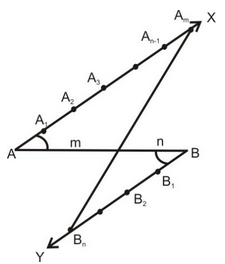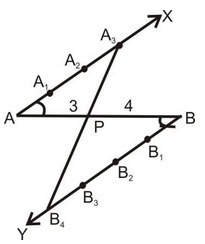# Alternative Method For Division Of A Line Segment Internally In A Given Ratio

## Constructions of Class 10

Use the following steps to divide a given line segment AB internally in a given ration m : n, where m and natural members.

### STEPS OF CONSTRUCTION:

(i) Draw a line segment AB of given length.

(ii) Draw any ray AX making an acute angle ∠BAX with AB.

(iii) Draw a ray BY, on opposite side of AX, parallel to AX making an angle ∠ABY equal to ∠BAX.

(iv) Mark off a points A1, A2,....Am’ on AX and n points B1, B2,...Bn on BY such that AA1 = A1A2 = ...... = Am-1 Am = B1B2 = ....Bn-1Bn.

(v) Join AmBn. Suppose it intersect AB at P.The point P is the required point dividing AB in the ratio m : n.

question 1. Decide a line segment of length 6 cm internally in the ratio 3:4.

Solution: Follow the following steps :### STEPS OF CONSTRUCTION:

(i) Draw a line segment AB of length 6 cm.

(ii) Draw any ray AX making an acute angle ∠BAX with AB.

(iii) Draw a ray BY parallel to AX by making ∠ABY equal to ∠BAX.

(iv) Mark of three point A1,A2,A3 on AX and 4 points B1, B2m B3, B4 on BY such that AA1 = A1A2 = A2A3 = BB1 = B1B2 = B2B3 = B2B4.

(v) Join A3B4. Suppose it intersects AB at a point P.

Then, P is the point dividing AB internally in the ratio 3:4.